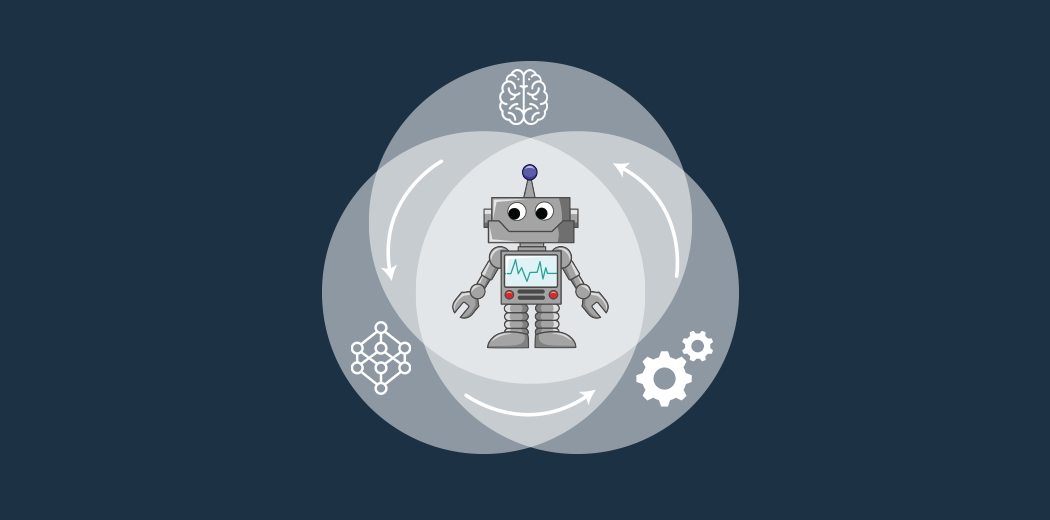Sreenath S — Published On November 28, 2020 and Last Modified On December 1st, 2020

This article was published as a part of the Data Science Blogathon.

## Introduction

Reinforcement Learning (RL) is a learning methodology by which the learner learns to behave in an interactive environment using its own actions and rewards for its actions. The learner, often called, agent, discovers which actions give the maximum reward by exploiting and exploring them.A key question is – how is RL different from supervised and unsupervised learning?

The difference comes in the interaction perspective. Supervised learning tells the user/agent directly what action he has to perform to maximize the reward using a training dataset of labeled examples. On the other hand, RL directly enables the agent to make use of rewards (positive and negative) it gets to select its action. It is thus different from unsupervised learning as well because unsupervised learning is all about finding structure hidden in collections of unlabelled data.

## Reinforcement Learning Formulation via Markov Decision Process (MDP)

The basic elements of a reinforcement learning problem are:

• Environment: The outside world with which the agent interacts
• State: Current situation of the agent
• Reward: Numerical feedback signal from the environment
• Policy: Method to map the agent’s state to actions. A policy is used to select an action at a given state
• Value: Future reward (delayed reward) that an agent would receive by taking an action in a given state

Markov Decision Process (MDP) is a mathematical framework to describe an environment in reinforcement learning. The following figure shows agent-environment interaction in MDP:

More specifically, the agent and the environment interact at each discrete time step, t = 0, 1, 2, 3…At each time step, the agent gets information about the environment state St. Based on the environment state at instant t, the agent chooses an action At. In the following instant, the agent also receives a numerical reward signal Rt+1. This thus gives rise to a sequence like S0, A0, R1, S1, A1, R2

The random variables Rt and St have well defined discrete probability distributions. These probability distributions are dependent only on the preceding state and action by virtue of Markov Property. Let S, A, and R be the sets of states, actions, and rewards. Then the probability that the values of St, Rt and At taking values s’, r and a with previous state s is given by,

The function p controls the dynamics of the process.

## Let’s Understand this Using an Example

Let us now discuss a simple example where RL can be used to implement a control strategy for a heating process.

The idea is to control the temperature of a room within the specified temperature limits. The temperature inside the room is influenced by external factors such as outside temperature, the internal heat generated, etc.

The agent, in this case, is the heating coil which has to decide the amount of heat required to control the temperature inside the room by interacting with the environment and ensure that the temperature inside the room is within the specified range. The reward, in this case, is basically the cost paid for deviating from the optimal temperature limits.

The action for the agent is the dynamic load. This dynamic load is then fed to the room simulator which is basically a heat transfer model that calculates the temperature based on the dynamic load. So, in this case, the environment is the simulation model. The state variable St contains the present as well as future rewards.

The following block diagram explains how MDP can be used for controlling the temperature inside a room:

## Limitations of this Method

Reinforcement learning learns from the state. The state is the input for policymaking. Hence, the state inputs should be correctly given. Also as we have seen, there are multiple variables and the dimensionality is huge. So using it for real physical systems would be difficult!